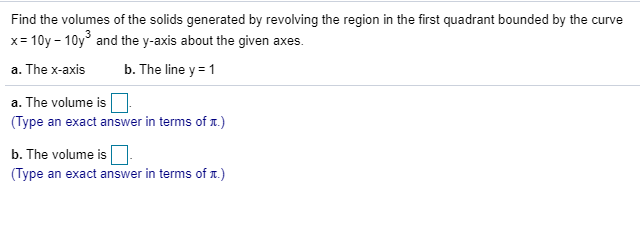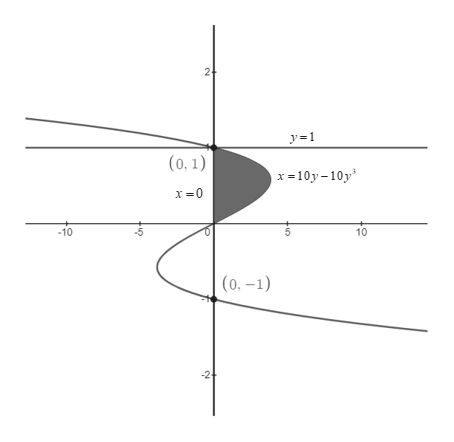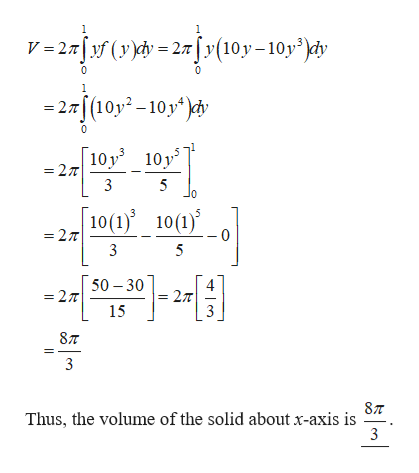# Find the volumes of the solids generated by revolving the region in the first quadrant bounded by the curvex= 10y - 10y° and the y-axis about the given axes.%3Da. The x-axisb. The line y = 1a. The volume is(Type an exact answer in terms of .)b. The volume is(Type an exact answer in terms of .)

Question
24 viewshelp_outlineImage TranscriptioncloseFind the volumes of the solids generated by revolving the region in the first quadrant bounded by the curve x= 10y - 10y° and the y-axis about the given axes. %3D a. The x-axis b. The line y = 1 a. The volume is (Type an exact answer in terms of .) b. The volume is (Type an exact answer in terms of .) fullscreen
check_circle

Step 1

The given curves are , x = 0 and y = 1.help_outlineImage Transcriptionclose2 y=1 (0, 1) x =10y -10y X =0 -5 10 -10 (0, –1) fullscreen
Step 2

a. Compute the volume of the shaded region of the solid a...help_outlineImage TranscriptioncloseV =2a[f (r)dv = 27[v(10y–10y )cy -2rj(t0y* - 10,")* = 27|(10y² – 10 y*)chv 10 y = 27 10y 3 5 10(1)' 10(1) = 27 3 5 50 – 30 4 = 27 = 27 15 87 3 87 Thus, the volume of the solid about x-axis is 3 fullscreen

### Want to see the full answer?

See Solution

#### Want to see this answer and more?

Solutions are written by subject experts who are available 24/7. Questions are typically answered within 1 hour.*

See Solution
*Response times may vary by subject and question.
Tagged in

### Other